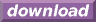#Calcute: freeware calculator

## Multiplication (mul, *)

A multiplication is the combination of two numbers resulting in one of them being added to its own value the number of times specified by the second number (which may be fractional). Calcute implements it using either the mul function or, equivalently, the * (product) operator.

```mul(3; 4)
12

3 * 4
12
```

In a multiplication, the value being multiplied is called the "multiplicand" and the value it is multiplied by is called the "multiplier". The result of an multiplication is called the "product".